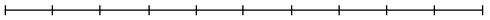### Home > CC2 > Chapter Ch5 > Lesson 5.1.1 > Problem5-9

5-9.

Find the missing values on the diagram below. Assume that each line is evenly divided.

 $\qquad \;a \qquad \qquad \qquad \qquad \qquad \qquad \qquad \quad \;\; 144\\ \; \overbrace{\qquad \qquad \;\;} \quad \qquad \qquad \qquad \qquad \;\; \overbrace{\qquad \qquad \qquad \qquad \quad}$$0\% \; \underbrace{\qquad} \qquad \qquad \qquad \qquad \qquad \quad \;\;\;\;\; \underbrace{\qquad \qquad \qquad \qquad \quad} \; 100\%\\ \quad \;\;\; 10\% \qquad \qquad \qquad \qquad \qquad \qquad \qquad \qquad \;\;\;\, c \\ \quad \; \; \underbrace{\qquad \qquad \qquad \qquad \quad \qquad}\\ \qquad \qquad \qquad \;\;\; b$

If the line is evenly divided, then each interval represents $10\%$ of the total value.
How many intervals are contained within $b$?

There are 5 intervals which means that $b$ represents $50\%$.

Follow the same method for $c$.

$144$ represents $40\%$ of the total value. So how much does $20\%$ represent?